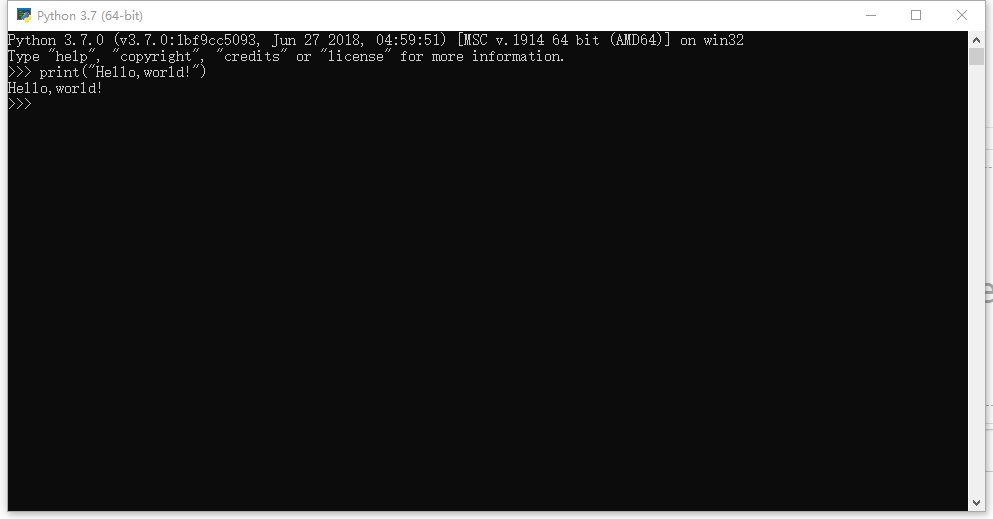Python的数据类型• 整型 (正数)
• 浮点型（小数）
• 布尔型（true|false）
• 字符串（用引号引起来）
• 数组（用中括号[]括起来）
• None（空值）
• 变量

整型a=3
b=8
c=a+b
d=a-b
e=a*b
f=a/b
g=b%a
print(a,b,c,d,e,f,g)a=true
b=true
c=a and b
print(c)

字符串

print("Hello,world!")

print("Hello,\"world\"!")

转义符的用法

• 转义本身符号，如引号、右斜线等
• 换行符：\n
• 制表符：\t

数组args=[1,1.25,"Hello",true,[1,2,3]]

数组中的元素怎么找？

agrs2=[[1,2,3],[4,5,6]]

变量发表评论1 条评论

1.小石 Yandex 19.3.1.828 Windows 10嘿嘿